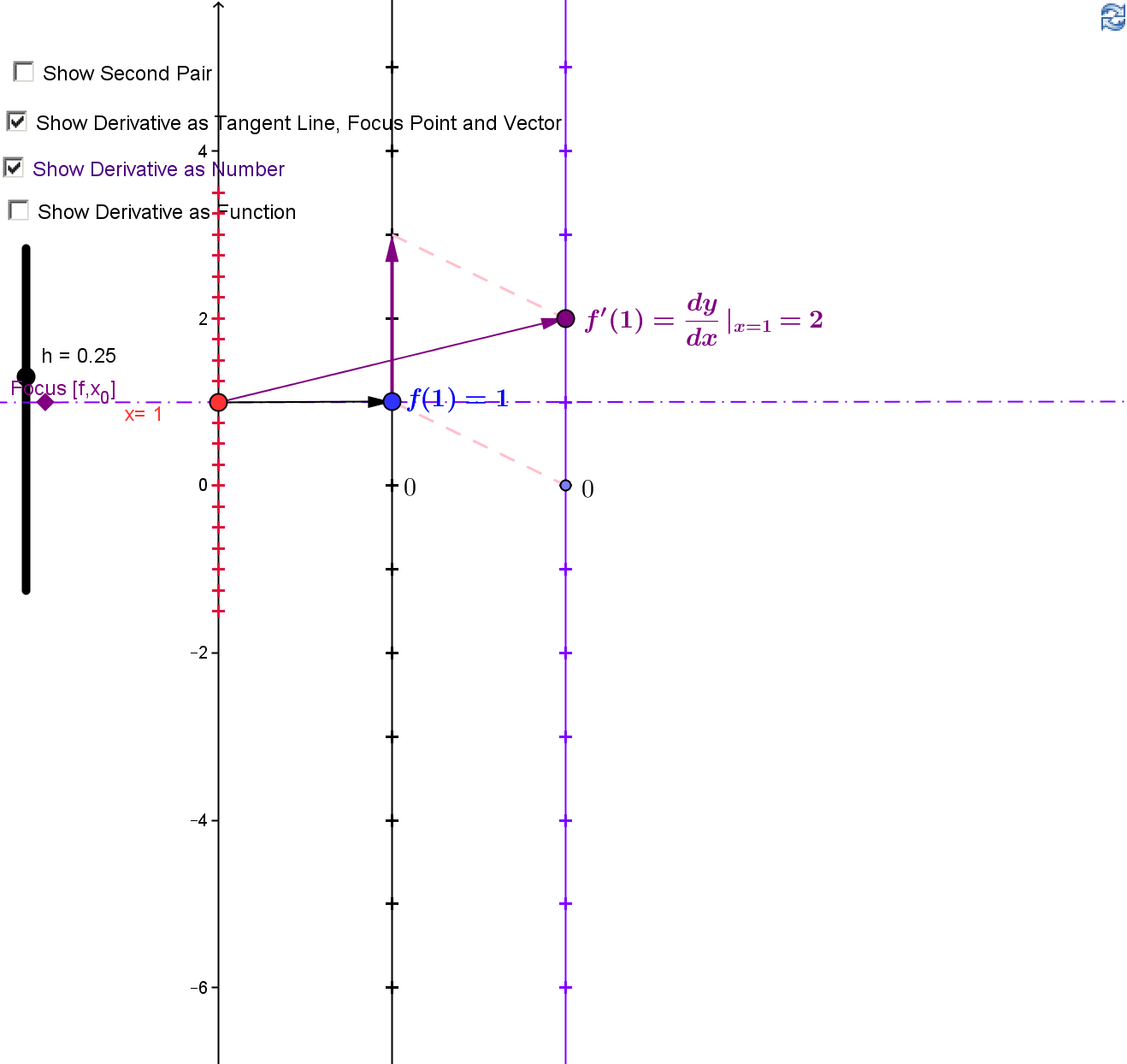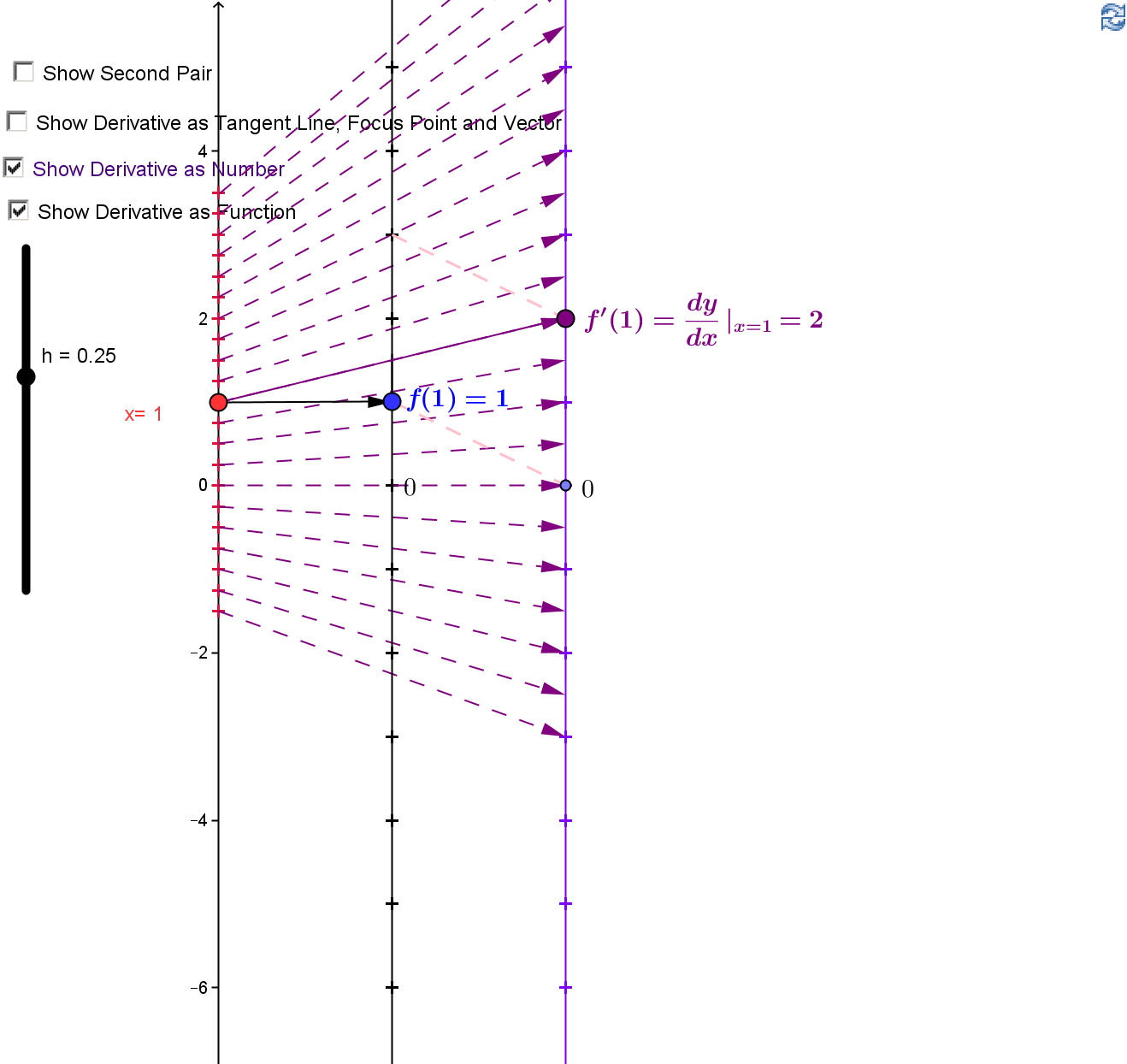The  derivative of $f$ is also a function, denoted $f'$, which is defined at $x$  whenever $f'(x)$ makes sense as a number, i.e. whenever $$f'(x) = \lim_{h \rightarrow 0} \frac {f(x+h)-f(x)}{h}$$ exists.
The derivative as a function is interpreted
1. The instantaneous velocity function for an object moving on a straight line;
2. The function that gives the slope of the tangent line at a point on the graph of the function;
3. The instantaneous rate of change function of a variable depending on an independent variable.
Visualizing the derivative function with mapping diagrams provides additional support to distinguish the information contained in the derivative as a function.Establish a separate parallel axis for measuring the derivative as a number. The derivative as a number is transferred from the vector visualization of the derivative on the target line to a number on this third axis created to record the values of the derivative. An arrow is drawn from the number on the domain axis to the number on the third axis indicating the derivative as a function.

Adding more arrows connecting values in the domain to the associated derivative values on the third axis visualizes the derivative function further. This supports understanding of the more traditional cartesian graph of the derivative where the vertical axis is used ambiguously for both function and derivative values  even though these values have separate  interpretations and units,The derivative function can be explored both with a mapping diagram and a  cartesian graph for different functions and at different points using the GeoGebra figure below.
Martin Flashman, 2 August 2015, Created with GeoGebra GGB 1
GGB 2